# Interpreting a line graph Online Quiz

#### Excel Pivot Tables and Dashboard. Simple & Quick tutorial!

Most Popular

7 Lectures 58 mins

#### Learn HTML Tables

15 Lectures 59 mins

#### Excel Analytics - Data Analysis with Pivot-Tables and Charts

46 Lectures 2.5 hours

Following quiz provides Multiple Choice Questions (MCQs) related to Interpreting a line graph. You will have to read all the given answers and click over the correct answer. If you are not sure about the answer then you can check the answer using Show Answer button. You can use Next Quiz button to check new set of questions in the quiz.Q 1 - The graph shows the number of visitors at a museum over six days. Find the weekday after which the number of visitors in a day started to increase for the second time?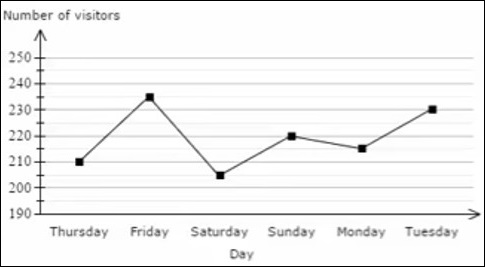### Explanation

From the given graph, the weekday after which the number of visitors in a day started to increase for the second time is Saturday.

Q 2 - The graph below shows the rainfall over a period of 12 months. Find the month after which the rainfall starting decreasing for the first time.### Explanation

From the graph, the month after which the rainfall starting decreasing for the first time is January.

Q 3 - The graph below shows noon temperatures for five days. What weekday was it when the noon temperature started to increase for the first time?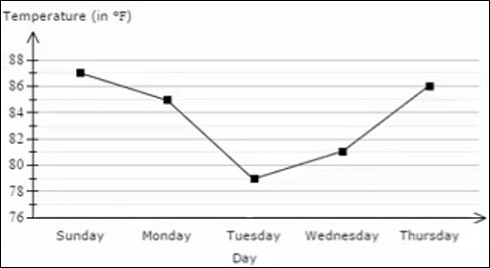### Explanation

From the graph, the weekday when the noon temperature started to increase for the first time was Tuesday.

Q 4 - The graph below shows the average hours of sun per day over a period of one year. What was the month in which there were the least number of average hours of sun per day?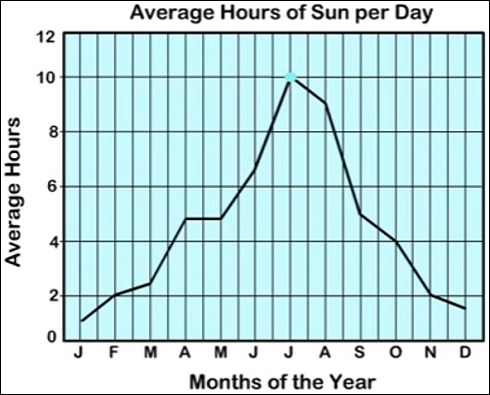### Explanation

From the graph, the month in which there were the second least number of average hours of sun per day was January.

Q 5 - The graph shows the number of visitors at a museum over six days. Find the weekday when there were the second least number of visitors in a day?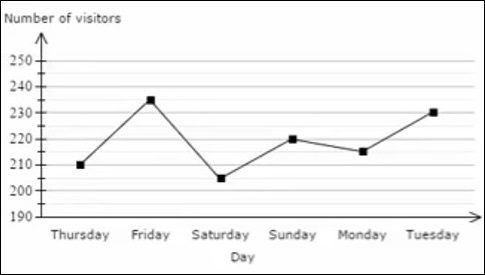### Explanation

From the graph, the weekday when there were the second least number of visitors in a day was Thursday.

Q 6 - The graph below shows noon temperatures for five days. What weekday had the second least noon temperature for the five days?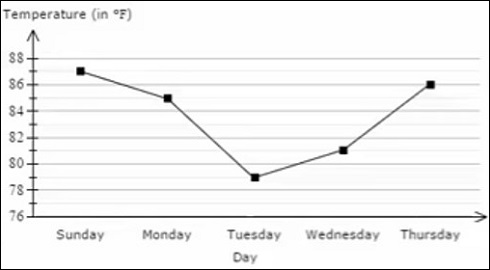### Explanation

From the graph, the weekday that had the second least noon temperature for the five days was Wednesday.

Q 7 - The graph below shows the average hours of sun per day over a period of one year. Which other month had the same number of average hours of sun per day as February?### Explanation

From the graph, the other month that had the same number of average hours of sun per day as February was November.

Q 8 - The graph below shows the average hours of sun per day over a period of one year. What was the month in which there were the most number of average hours of sun per day?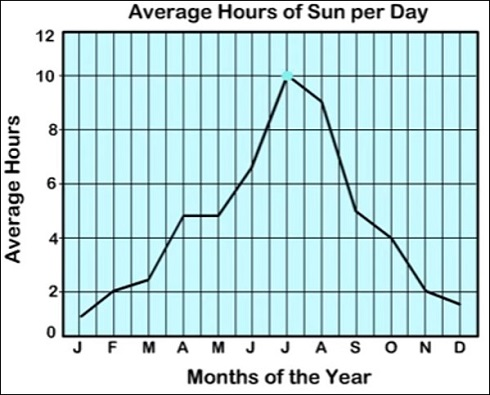### Explanation

From the graph, the month in which there were the most number of average hours of sun per day was July.

Q 9 - The graph below shows the rainfall over a period of 12 months. Find the month in which there was the second least rainfall.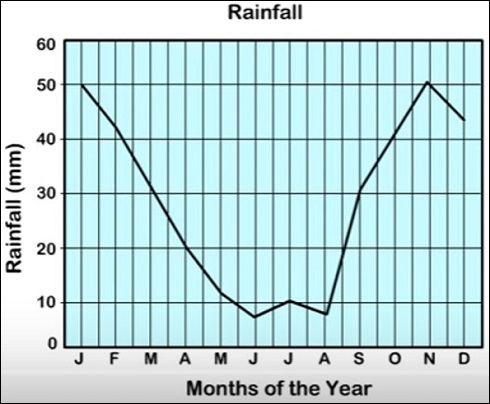### Explanation

From the graph, the month in which there was the second least rainfall was August.

Q 10 - The graph shows the number of visitors at a museum over six days. Find the weekday when there were the greatest number of visitors in a day?### Explanation

From the graph, the weekday when there were the greatest number of visitors in a day was Friday.

interpreting_line_graph.htm